# Issn: 2277-9655 [Jain* et alISSN: 2277-9655 [Jain* et al., 5(11): November, 2016] Impact Factor: 4.116 IC™ Value: 3.00 CODEN: IJESS7IJESRT International Journal of Engineering Sciences & Research Technology HIGH ACCURACY FACE REORGANIZATION BY PCA - SVD Rahul Jain*, Sanjay Kumar * M.Tech Scholar Jaipur National University, Jaipur Associate Professor CSE Deptt, Jaipur National University , Jaipur DOI: 10.5281/zenodo.164897 ABSTRACT Face Detection or Reorganization is a Part of biomedical technology which is working for the many applications like Banking, Human-Computer interaction, Implementation Security, Retrieval of Data Base, etc. Base paper is using Principal Component Analysis (PCA) for Face Detection. PCA is a methodology for the Face Detection which is using for reducing the variable in the Face Reorganization. The image in the training set can be represented as linear combination of Eigen Vector which is called by "Eigen Faces". From the Covariance Matrix, we can get the Eigen Vectors of training Image set called the basic function. For Face Reorganization in PCA method, the test image is projected onto subspace with the help of Eigen Faces and the for Face Reorganization. Distance will measure such as Euclidean distance. All the test images will check by this method for Face Reorganization. In this Research , we are improving the Accuracy of the Face reorganization system by use PCA+SVD .We are taking the training data sets of different faces for recognize the face . From the result session, we can see the Accuracy of face detection . Accuracy is getting increase as we apply PCA+SVD. KEYWORDS: Face Recognition, Principle Component Analysis (PCA), Eigenface, Covariance matrix, Face database. INTRODUCTION A facial recognition system is computer software developed particularly for the recognition & verification of a person from a digitized picture from source of video. One method to do this is by making comparison in choosing facial attributes from an image & facial database. It is generally implemented in security systems & can be put in contrast to other relative biometrics systems like fingerprints or iris identification systems . RELATED WORK From Year of 2000, a rapid growth in the field of hardware & software technology tends to develop fancy & friendly user interfaces & even more possible than that. As an illustration, for some of the patients that are unable to type or use a mouse, the eve gaze systems are developed for them. In such systems user is able to control the functions of mouse by looking at picture or word as shown on the screen. In the year 2007, Sony launched its first camera for users named as Cyber-shot DSC T20 having a function of smile shutter. This feature is able to recognize three faces of humans to maximum in the picture & automatically click the picture is it detects a smile. Several users complained about this feature to be lacking in terms of accuracy & it is found that the Sony smile shutter can detect only big smiles but not slight smile. Also, it will be triggered if teeth of the individuals appear. So, we suggest a better smile detecting system that is more accurate & can be used on a personal computer & web-cam. The issues linked to detection of smile are covered by face expression recognition. There are a lot of academic researchers conducted over facial expression recognition, like  & , but not deep research is done over detection of smile. Though, rate of detection & algorithm of Sony’ smile shutter are not provided. A smile measuring software is recently release by sensing Component Company named Omron . It is able to identify the faces or one or more than one person & allocate a smile factor of 0%-100%. Omron makes use of a 3-D face mapping technique & acclaim that it has detection rate of more than 90%. Though, it is not available & we are unable to test its performance. So, we will be testing our program with Sony T300 & exlain that we have better performance for recognizing slight smiles & minimize rate of false alarm on grimace expressions. PCA ALGORITHM The PCA (Principal component analysis) is the popular methods for to reduce face recognition variables. In the PCA, the faces are presented as the linear combination of the weighted eigen-vectors called as Eigen faces ; Such eigenvectors are gained from matrix covariance a training image set called as the basis function. The Eigen faces number which obtained may be equal to image numbers in a training set. The eigen faces take the advantage in between the pixels that among the images in the dataset employing the covariance matrix. Such Eigen vectors are characterized the new face space where the images get represented. To alter the required documentation, let us introduce the following symbol. Assume that the training image set-I consist of the N images each one having the size a×b pixels. Hence, by using the conventional method of row appending converts every image into the column vector of dimension a×b. I ={ i1, i2 , ........, in } ......(1) The covariance matrix ‘c’ of the training image set is considered by using equation (3.2)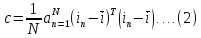The Eigenvectors finds that ‘U’ have an appearance of a face, they’re called the Eigen faces. Generally, they called as the Ghost Images, due to their weird appearance. And after a face space has constructed, the linear combination of a feature vector is formed by the eigenvectors of a covariance matrix. Project the face space image with the following equation.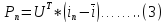Here, Pn, n= 1, 2,….., N are weights of a vector that associated with eigenvectors in ‘c.' One can try different things with eigenvectors quantity to process the weights, for the most part, just a couple of amount give adequate data to sufficiently representing the image to a face space. For an acknowledgment of unknown face or the test picture, standardize it by subtracting from the mean vector of entire images in a set. At that point by utilizing condition (4) project a standardized test image as appeared in the accompanying condition,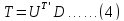Where, D - normalized test image. And after a feature vector/weight vector test image has discovered, the next-step is to characterize it. For the task of classification, we could utilize Euclidean distance classifier. e = min||T- Pn|| ......(5) N=1,2,…..N. On the off chance that the separation is little i.e. small distance, we can say that the images are comparable, and we are able to choose a most comparative image in a database. PROBLEM STATEMENT The face recognition is the biometric technology having the vast range of the potential applications likes database retrieval, virtual reality, human-computer interaction, information security, banking, and access control, etc. Hence, by using the PCA (Principal Component Analysis) a base paper addresses the face recognition system building. The recognition is functioned by the new image projection (test image) on a sub-space that spanned by eigen faces and then the classification is done by the methods of distance measure like the Euclidean distance. Many experiments have been done for performance evaluation a face recognition system. In a base paper, the PCA algorithm is given that based on face detection. And for particular research, we find an Accuracy issue. The Face Detection Accuracy may be further increased by suggested methodology. PROPOSED METHODOLOGY The PCA (Principal Component Analysis) is the statistical method that used for dimension recognition and reduction, & the broadly used for a facial feature of recognition and extraction. The PCA is known as the ESP (Eigen Space Projection) that has depended on linear projection an image space in order to low Dimension feature space which is known as the Eigen space. Many PCA -based systems of face recognition have been developed in the last decade. However, an existing faces recognition systems (PCA -based) are hard measure due to computational memory and cost needing load. The 2- D (Two Dimensional) facial image is represented as the 1- D (dimensional) vector by concatenating every column or row into the long thin vector. Let’s assume, and we have N Size M vectors (= rows of the image £ columns of the image) illustrating a sampled images set. Pj’s represent the pixel Values.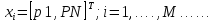(6) And by subtracting a mean image from every image vector, the images become the mean centered. Let, ‘m’ stand for a mean image.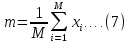And suppose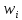be the defined as the mean centered image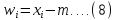The aim is to find the set of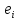’s that have the greatest possible Projection on to every of’s. The singular value de-composition is the linear algebra output. And SVD in the digital applications gives robust/strong technique large image storage as smaller one, as well more manageable square ones. The matrix A singular value de-composition of matrix m x n is also given in a form,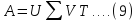Where, the U = m x n orthogonal matrix; size m x n; V = n x n orthogonal matrix, and Σ = m x n matrix consisting a singular value of the A along its most important diagonal. The SVD (Singular value decomposition) is a suitable rectangular matrix factorization which has been used largely in the data retrieval for minimizing document dimension vector space. The de-composition may be defined as the follows. Given is the generic rectangular n×m matrix- A, its SVD is: A = U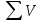T .... (10) Where U = n × r matrix; VT = r × m matrix And, Σ = r x r diagonal matrix. The two- matrices U & V are unitary, that is., U T U= I & V T V= I. The diagonal components of Σ are of singular values like δ1 > δ2> δr >0. Where r = rank of matrix A. The SVD utilize a linear combination of columns and rows of A for decomposition.The initial trivial method of using SVD as the unsupervised quality reduction is following. The given ‘E’ as the training set for examples it is represented in the feature-space of the n- features, We may notice it as the matrix, that is, an examples sequence E = (e 1....e m ). With the SVD, the n×m matrix E may factorize as the E= U Σ V T. The factorization implies that we are able to concentrate the learning problem on the brand new space by using the given transformation by the U matrix. It is a new space that is illustrated by a matrix: E' = U T E = Σ V T ..... (11) Where the every example is observed with the ‘r’ new features. Every new feature is gained as the linear combination of original features, that is, every feature vector el may be seen as the new feature vector el'= UT el. When the end feature space is vast, whereas the trailing set cardinality is small, the is, n>>m, the SVD application results in the original feature reduction space as a rank ‘r’ of a matrix E is ‘r’ < min (n, m). The most interesting technique of utilizing SVD is like the selection of un-supervised feature to exploit an approximated computation, that is: A ⸗ Ak = U m *k Σ k*k V T k*n .....(12) Where, ‘k’ = Smaller than a rank r. The essential step in the direction of denoising is to obtain SVD. In order to get the SVD of an image ‘A,' of the size [MxN], A is re-shaped to obtain the matrix A1 of a size [4 x MN/4]. The SVD of A1 got as, [U S]= SVD (A1) ....(13) Share with your friends: